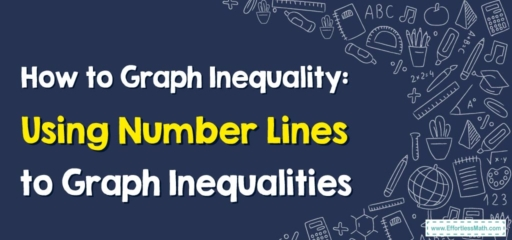# How to Graph Inequality: Using Number Lines to Graph Inequalities

Today, we're merging algebra and geometry as we explore how to use number lines to graph inequalities. This technique allows us to visually represent the solution to an inequality and better understand the relationship between the variables.## 1. Understanding Inequalities on a Number Line

An inequality can be graphed on a number line to show all its possible solutions. For example, if $$x > 2$$, this means that x can be any number greater than $$2$$. On a number line, we represent this by a ray pointing to the right from $$2$$.

## 2. Graphing Inequalities on a Number Line

Graphing inequalities on a number line is a straightforward process once you’ve solved the inequality.

## Step-By-Step Guide to Using Number Lines to Graph Inequalities

Let’s dive into the step-by-step process.

### Step 1: Solve the Inequality

First, solve the inequality to find the range of solutions.

### Step 2: Draw the Number Line

Draw a number line that includes the value of your solution and a few numbers on either side.

### Step 3: Mark the Solution on the Number Line

Place an open circle at your solution value on the number line if your inequality is “$$>$$” or “$$<$$”. Use a closed or filled circle if your inequality includes “$$≥$$” or “$$≤$$”.

### Step 4: Shade on the Correct Side

Shade to the right if your inequality is “$$>$$” or “$$≥$$”. Shade to the left if it is “$$<$$” or “$$≤$$”. This shading represents all possible solutions to the inequality.

For example, if we have the inequality $$x > 2$$:

1. Solve it: $$x > 2$$.
2. Draw the number line from $$0$$ to $$4$$.
3. Place an open circle at $$2$$.
4. Shade everything to the right of $$2$$.

Graphing inequalities on a number line might seem like a small skill, but it’s an essential part of understanding and working with inequalities. It’s another great tool in your math toolkit.

As always, keep practicing, stay curious, and enjoy your mathematical journey!

This blog post has introduced the concept of graphing inequalities on a number line and provided a step-by-step guide to this process. With some practice, this method will become second nature. Happy graphing!

### What people say about "How to Graph Inequality: Using Number Lines to Graph Inequalities - Effortless Math: We Help Students Learn to LOVE Mathematics"?

No one replied yet.

X
30% OFF

Limited time only!

Save Over 30%

SAVE $5 It was$16.99 now it is \$11.99